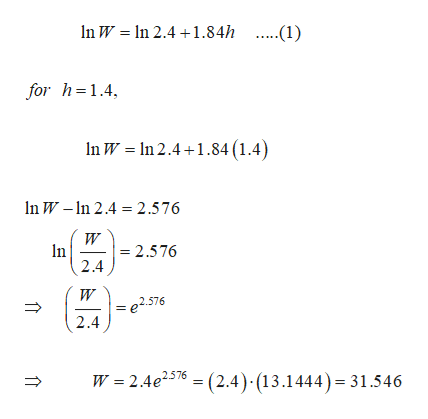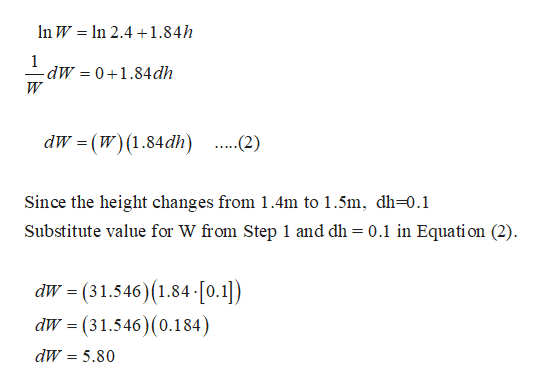# For children between the ages of 5 and 13 years, the Ehrenberg equationln W = ln 2.4 + 1.84hgives the relationship between the weight W (in kilograms) and the height h (in meters) of a child. Use differentials to estimate the change in the weight of a child who grows from 1.4 m to 1.5 m. (Round your answer to two decimal places.)

Question

For children between the ages of 5 and 13 years, the Ehrenberg equation

ln W = ln 2.4 + 1.84h

gives the relationship between the weight W (in kilograms) and the height h (in meters) of a child. Use differentials to estimate the change in the weight of a child who grows from 1.4 m to 1.5 m. (Round your answer to two decimal places.)

check_circleExpert Solution
Step 1

Given data;help_outlineImage Transcriptionclosen W n 2.41.84h .....(1) for h=1.4 In W In 2.4+1.84 (1.4) n W-n 2.4 = 2.576 W In 2.576 2.4 = e2576 2.4 W 2.4e2576(2.4) (13.1444) 31.546 fullscreen
Step 2

Differentiate equation ...help_outlineImage TranscriptioncloseIn W In 2.41.84h 1 -dW 0+1.84dh W dW (W) (1.84dh) ...(2) Since the height changes from 1.4m to 1.5m, dh-0.1 Substitute value for W from Step 1 and dh = 0.1 in Equati on (2) dW (31.546) (1.84 [0.1]) dW (31.546) (0.184) dW 5.80 fullscreen

### Want to see the full answer?

See Solution

#### Want to see this answer and more?

Solutions are written by subject experts who are available 24/7. Questions are typically answered within 1 hour*

See Solution
*Response times may vary by subject and question
Tagged in

### Derivative